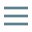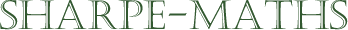Previous topic:
### Factorising quadratics when $a \ne 1$
The general quadratic form is $ax^2 \pm bx \pm c$ where $a, b, c \in\Bbb{R}$ ($a, b, c$ are in the set of real numbers). Up to this point, we have only attempted to factorise quadratics where $a=1$. In this section, we will look at quadratics where $a$ is other than $1$.
The methods available to us are many and various. I have a particular favourite, as it enabled me, slow as I am, to factorise these things in my head as easily as when $a=1$. However, I will cover several methods and you should use the one that works best for you.